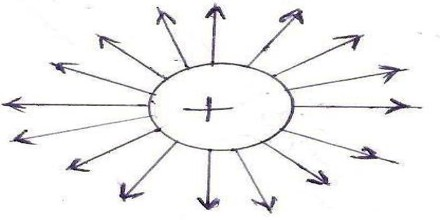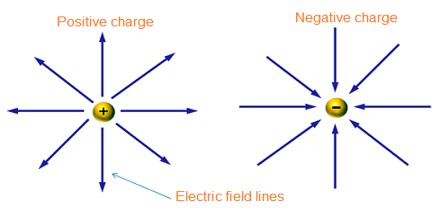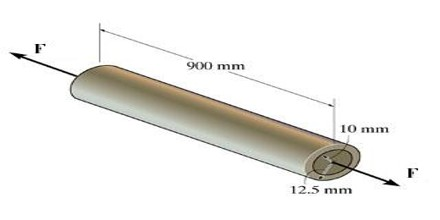Line of Force

Definition

Line of Force is an imaginary line in a field of force, whose tangent at any point gives the direction of the field at that point and whose number through unit area perpendicular to the field represents its intensity. In physics, Line of Forc is a path followed by an electric charge free to move in an electric field or a mass free to move in a gravitational field, or generally any appropriate test particle in a given force field.Lines of Force are lines in any such force field the tangent of which at any point gives the field direction at that point and the density of which gives the magnitude of the field. The concept of lines of force was introduced into physics in the 1830s by the English scientist Michael Faraday, who considered magnetic and electric effects in the region around a magnet or electric charge as a property of the region rather than an effect taking place at a distance from a cause. His theory predicts that electricity, light, and gravity have finite propagation delays. The theories and experimental data of later scientific figures such as Maxwell, Hertz, Einstein, and others are in agreement with the ramifications of Faraday’s theory. Nevertheless, Faraday’s theory remains distinct. Unlike Faraday, Maxwell and others (e.g., J.J. Thomson) thought that light and electricity must propagate through ether. In Einstein’s relativity, there is no ether, yet the physical reality of force is much weaker than in the theories of Faraday.Properties of Electric Lines of Forces

Electric lines of force can be defined as a way or path, it may be straight or curved, so that the tangent at any point to it gives the direction of the electric field intensity at that point. The electric lines of force that represent the field of a positive electric charge in space consist of a family of straight lines radiating uniformly in all directions from the charge where they originate. A second positive charge placed in the field would travel radially away from the first charge.

• The direction of Electric field intensity at a point can be calculated by drawing the tangent to the line of force at that point.
• Due to the attraction between unlike charges, the electric lines of force reduce longitudinally.
• Due to the repulsion between the like charges, the line of force applies a lateral pressure.
• Electric lines of forces never intersect each other.
• All the components of electric field intensity are perpendicular to the outermost layer of the conductor.Tube of Force

A tube of electrostatic induction or field tube also called a tube of force are the lines of electric force which moves so that its beginning traces a closed curve on a positive surface, its end will trace a corresponding closed curve on the negative surface, and the line of force itself will generate an inductive tubular surface. Such a tube is called a “Solenoid”. There is a pressure at right angles to a tube of force of one half the products of the dielectric and magnetic density. If through the growth of a field the tubes of force are spread sideways or in width there is a magnetic reaction to that growth in intensity of electric current. However, if a tube of force is caused to move endwise there is little or no drag to limit velocity. Tubes of force are absorbed by bodies imparting momentum and gravitational mass. Tubes of force are a group of electric lines of force.

Maxwell used this term for Faraday’s line of forces while expressing his fluidic assumptions involved in his mathematization of Faraday’s theories.